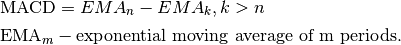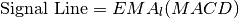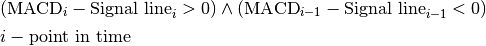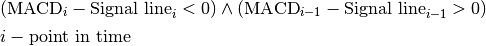# MACD Strategy

## Description

MACD Strategy is a strategy build to gain profit on alternately buying and selling shares. MACD Strategy calculates two lines of values:

• MACD line – difference between the n-period EMA and the k-period EMA• Signal line – The l-period EMA of the MACDIn the moment when the MACD line crosses below MACD Signal line then it is a signal to sell shares and respectively when it crosses above MACD Signal line it is a time to buy shares.

## Parameters

PARAMETER NAME DESCRIPTION ESSENTIAL
Length of Short-term Average Number of last ‘last trades’ events used to compute short-term EMA for MACD Yes
Length of Long-term Average Number of last ‘last trades’ events used to compute long-term EMA for MACD Yes
Length of MACD Signal Line Number of last values of MACD line used to compute value of MACD signal line Yes

## Conditions

### Open position

Amount One asset per order
Price Last market price
Type Price limitIf MACD line crosses above Signal line then it is a signal to buy.

### Close position

Side Sell
Amount One asset per order
Price Last market price
Type Price limitIf MACD line crosses below Signal line then it is a signal to sell.

### Termination

There is no strategy termination condition.

### Time frame

Strategy bases on two lines which are functions of some number (equal to number of periods) of prices. Frequency of new positions depends on the price values, EMA calculations and the number of periods.